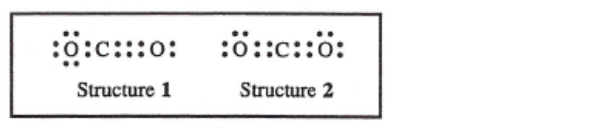# Problem: These two electron-dot formulas for carbon dioxide (given below) both satisfy the octet rule, but one is preferred over the other. Which of the statements identifies the preferred structure and also the reason it is preferred?a) Structure 1 is preferred because the triple bond is stronger than the double bond.b) Structure 1 is preferred because the formal charge on carbon is negative. c) Structure 2 is preferred because the formal charge on each atom is zero.d) Structure 2 is preferred because the bonds are equal.

###### FREE Expert Solution
86% (371 ratings)
###### FREE Expert Solution

We’re being asked to determine the preferred structure for carbon dioxide (CO2)

For this problem, we need to compare the formal charge of each structure.

Recall that the formula for the formal charge is:

86% (371 ratings)###### Problem Details

These two electron-dot formulas for carbon dioxide (given below) both satisfy the octet rule, but one is preferred over the other. Which of the statements identifies the preferred structure and also the reason it is preferred?

a) Structure 1 is preferred because the triple bond is stronger than the double bond.

b) Structure 1 is preferred because the formal charge on carbon is negative.

c) Structure 2 is preferred because the formal charge on each atom is zero.

d) Structure 2 is preferred because the bonds are equal.What scientific concept do you need to know in order to solve this problem?

Our tutors have indicated that to solve this problem you will need to apply the Formal Charge concept. You can view video lessons to learn Formal Charge. Or if you need more Formal Charge practice, you can also practice Formal Charge practice problems.

What is the difficulty of this problem?

Our tutors rated the difficulty ofThese two electron-dot formulas for carbon dioxide (given be...as medium difficulty.

How long does this problem take to solve?

Our expert Chemistry tutor, Jules took 3 minutes and 38 seconds to solve this problem. You can follow their steps in the video explanation above.

What professor is this problem relevant for?

Based on our data, we think this problem is relevant for Professor Hashim's class at UCF.